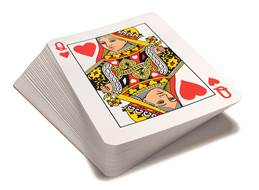# Drawing a Card

Go back to  'Probability'

Consider a standard deck of playing cards:As you know, any such deck has 52 cards, with 13 cards each from the following four suits: Diamonds, Hearts, Clubs and Spades. The suits Diamonds and Hearts are of color red, while the suits Clubs and Spaces are of color black.

Consider as your random experiment the drawing of a card from this deck. The sample space has 52 outcomes in this case.

Suppose that the deck is well-shuffled. In such a scenario, no outcome will be particularly preferred over another outcome. Thus, if the experiment is repeated a large number of times (if you draw a card a large number of times, with the deck being well-shuffled each time), then the relative occurrence of any one particular outcome will approach $$\frac{1}{{52}}$$. Thus, the probability of each outcome of the sample space is $$\frac{1}{{52}}$$.

Note that from the perspective of probability, a well-shuffled deck is analogous to a fair coin or a fair, non-loaded die. For all three, each outcome in the sample space is equally likely.

Now, for a well-shuffled deck, suppose that you define an event E as drawing a red card, and an event F as drawing a card of Spades. Event E has 26 possible outcomes (13 Diamonds + 13 Hearts), while event F has 13 outcomes (the 13 cards of Spades). Thus,

\begin{align}&P\left( E \right) = \frac{{{\rm{No}}{\rm{. of \,outcomes \,\,favorable \,to\, }}E}}{{{\rm{No}}{\rm{. of \,total \,\,outcomes}}}}\\\,\,\,\,\,\,\,\,\,\,\,\,\, &\qquad\;\;= \frac{{26}}{{52}} = \frac{1}{2}\\&\;P\left( F \right) = \frac{{{\rm{No}}{\rm{. of \, outcomes\,\, favorable \,to \,}}F}}{{{\rm{No}}{\rm{. of \,total \,\, outcomes}}}}\\\,\,\,\,\,\,\,\,\,\,\,\,\, &\qquad\;\;\;= \frac{{13}}{{52}} = \frac{1}{4}\end{align}

Example 1: A card is drawn from a well-shuffled deck. Find the probability of the card either being red or a King card.

Solution: Let us define the event E as: the card drawn is either red or a King card. How many outcomes are there which are favorable to E? There are 26 red cards, and 4 cards which are Kings. However, 2 of the red cards are themselves Kings. If we add 26 and 4, we will be counting these two cards twice. Thus, the correct number of outcomes which are favorable to E is

$26 + 4 - 2 = 28$

The probability of E occurring will be

\begin{align}&P\left( E \right) = \frac{{{\rm{No}}{\rm{. of \,outcomes\,\, favorable \,to\, }}E}}{{{\rm{No}}{\rm{. of \,total \,\,outcomes}}}}\\\,\,\,\,\,\,\,\,\,\,\,\,\, &\qquad\;\;= \frac{{28}}{{52}} = \frac{7}{{13}}\end{align}

Probability
Probability
grade 10 | Questions Set 2
Probability